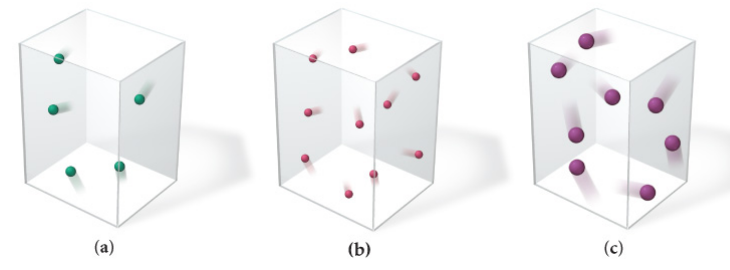# Problem: Which gas sample has the greatest pressure? Assume that all the samples are at the same temperature. Explain.a) PV = nRT: Because V and T are constant, P prop. to n. The sample with the smallest number of gas particles will have the highest P.  Pb &gt; Pc &gt; Pab) PV = nRT: Because P and n are constant, V prop to T. The sample with the largest number of gas particles will have the highest P.  Pb &gt; Pc &gt; Pac) PV = nRT: Because V and T are constant, P prop to n. The sample with the largest number of gas particles will have the highest P.  Pa &gt; Pc &gt; Pbd) PV = nRT: Because V and T are constant, P prop to n. The sample with the largest number of gas particles will have the highest P.  Pb &gt; Pc &gt; Pa

###### FREE Expert Solution
79% (136 ratings)###### Problem Details

Which gas sample has the greatest pressure? Assume that all the samples are at the same temperature. Explain.a) PV = nRT: Because V and T are constant, P prop. to n. The sample with the smallest number of gas particles will have the highest P.  Pb > Pc > Pa

b) PV = nRT: Because P and n are constant, V prop to T. The sample with the largest number of gas particles will have the highest P.  Pb > Pc > Pa

c) PV = nRT: Because V and T are constant, P prop to n. The sample with the largest number of gas particles will have the highest P.  Pa > Pc > Pb

d) PV = nRT: Because V and T are constant, P prop to n. The sample with the largest number of gas particles will have the highest P.  Pb > Pc > Pa